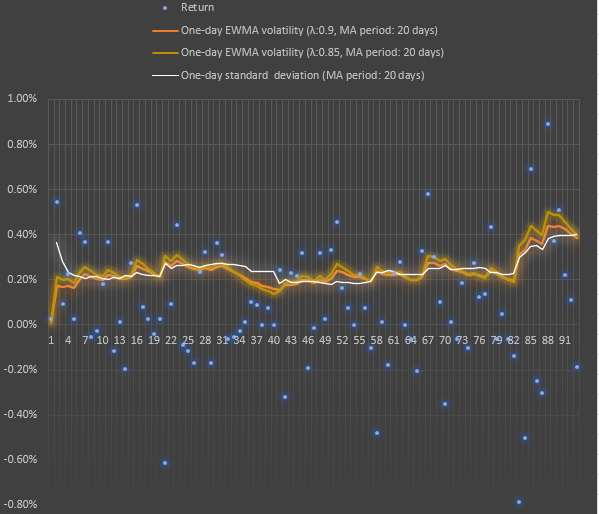Exponential Weighted Volatility (EWMA, Exponential Weighted Moving Average) is an approach to volatility calculation. The main objective of the EWMA approach is to closely track the volatility as it changes.

The purpose of weighting is to have a faster adjustment of calculated volatility by giving more weight to the latest observations:

\begin{equation}\sigma_t^2 = \lambda\sigma_{t-1}^2 + (1-\lambda)r_{t-1}^2\label{eq1}\end{equation}

Where:

σt and σt-1  — EWMA volatility of return at time t and EWMA volatility of return at time t-1

rt-1 — return at time t-1

λ — weight coefficient (so, you have weight λ-1 for the latest observation of return and λ for the previous EVMA).

For daily volatility, RiskMetrics Group (the guys who initially published the EWMA volatility model) uses EWMA with λ=0.94. In fact, other lambda values can be chosen based on your timeframe and purposes (you need slow or you need fast volatility tracking). The lambda can be also set as a function of the latest return observations. Reasonable values for lambda are in the range from 0.80 to 0.98.

For risk-management purposes, VaR based on EWMA volatility is more useful, compared to VaR based on standard deviation. Additionally, the EWMA approach requires relatively little data to store: to update the estimate, we only need a prior estimate of the variance rate and the most recent observation value.As you can see in the example above, EWMA-based volatility can be higher or lower than the standard deviation of the same timeframe.

Here is a VBA code for the EWMA variance function:

'-------------------------------------------------------------------------
' Source: risksir.com
' Description: Calculation of EWMA-based variance
'-------------------------------------------------------------------------

Function EWMA(iReturns As Range, iLambda As Single) As Double
Dim PeriodMA As Integer
PeriodMA = iReturns.Rows.Count

ReDim iU2(PeriodMA) As Double
For i = 1 To PeriodMA
iU2(i) = (iReturns(i).Value) ^ 2
Next i

ReDim iWeight(PeriodMA) As Single
iWeight(PeriodMA) = 1 - iLambda
For i = 1 To (PeriodMA - 1)
iWeight(PeriodMA - i) = iWeight(PeriodMA - i + 1) * iLambda
Next i

ReDim iWeightedU2(PeriodMA) As Single
For i = 1 To PeriodMA
iWeightedU2(i) = iU2(i) * iWeight(i)
Next i

EWMA = 0
For i = 1 To PeriodMA
EWMA = EWMA + iWeightedU2(i)
Next i

End Function